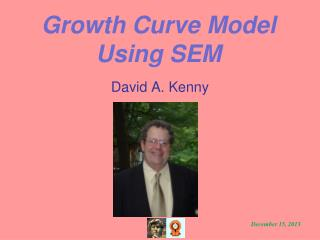DownloadDownload PresentationGrowth Curve Model Using SEM

# Growth Curve Model Using SEM

Download Presentation## Growth Curve Model Using SEM

- - - - - - - - - - - - - - - - - - - - - - - - - - - E N D - - - - - - - - - - - - - - - - - - - - - - - - - - -
##### Presentation Transcript

1. Growth Curve Model Using SEM David A. Kenny

2. Thanks due to Betsy McCoach

3. Linear Growth Curve Models • We have at least three time points for each individual. • We fit a straight line for each person: • The parameters from these lines describe the person. • Nonlinear growth models are possible.

4. The Key Parameters • Slope: the rate of change • Some people are changing more than others and so have larger slopes. • Some people are improving or growing (positive slopes). • Some are declining (negative slopes). • Some are not changing (zero slopes). • Intercept: where the person starts • Error: How far the score is from the line.

5. Latent Growth Models (LGM) • For both the slope and intercept there is a mean and a variance. • Mean • Intercept: Where does the average person start? • Slope: What is the average rate of change? • Variance • Intercept: How much do individuals differ in where they start? • Slope: How much do individuals differ in their rates of change: “Different slopes for different folks.”

6. Measurement Over Time • measures taken over time • chronological time: 2006, 2007, 2008 • personal time: 5 years old, 6, and 7 • missing data not problematic • person fails to show up at age 6 • unequal spacing of observations not problematic • measures at 2000, 2001, 2002, and 2006

7. Data • Types • Raw data • Covariance matrix plus means Means become knowns: T(T + 3)/2 Should not use CFI and TLI (unless the independence model is recomputed; zero correlations, free variances, means equal) • Program reproduces variances, covariances (correlations), and means.

8. Independence Model in SEM • No correlations, free variances, and equal means. • df of T(T + 1)/2 – 1

9. Specification: Two Latent Variables • Latent intercept factor and latent slope factor • Slope and intercept factors are correlated. • Error variances are estimated with a zero intercept. • Intercept factor • free mean and variance • all measures have loadings set to one

10. Slope Factor • free mean and variance • loadings define the meaning of time • Standard specification (given equal spacing) • time 1 is given a loading of 0 • time 2 a loading of 1 • and so on • A one unit difference defines the unit of time. So if days are measured, we could have time be in days (0 for day 1 and 1 for day 2), weeks (1/7 for day 2), months (1/30) or years (1/365).

11. Time Zero • Where the slope has a zero loading defines time zero. • At time zero, the intercept is defined. • Rescaling of time: • 0 loading at time 1 ─ centered at initial status • standard approach • 0 loading at the last wave ─ centered at final status • useful in intervention studies • 0 loading in the middle wave ─ centered in the middle of data collection • intercept like the mean of observations

12. Different Choices Result In • Same • model fit (c2 or RMSEA) • slope mean and variance • error variances • Different • mean and variance for the intercept • slope-intercept covariance

13. some intercept variance, and slope and intercept being positively correlated no intercept variance intercept variance, with slope and intercept being negatively correlated

14. Identification • Need at least three waves (T = 3) • Need more waves for more complicated models • Knowns = number of variances, covariances, and means or T(T + 3)/2 • So for 4 times there are 4 variances, 6 covariances, and 4 means = 14 • Unknowns • 2 variances, one for slope and one for intercept • 2 means, one for the slope and one for the intercept • T error variances • 1 slope-intercept covariance

15. Model df • Known minus unknowns • General formula: T(T + 3)/2 – T – 5 • Specific applications • If T = 3, df = 9 – 8 = 1 • If T = 4, df = 14 – 9 = 5 • If T = 5, df = 20 – 10 = 10

16. Three-wave Model • Has one df. • The over-identifying restriction is: M1 + M3 – 2M2 = 0 (where “M” is mean) i.e., the means have a linear relationship with respect to time.

17. Intercept Factor